# Spatial Data Types¶

Backendless database supports the following spatial data types:

• `POINT` - represents a single point/location in coordinate space. For the points representing locations on a map, the coordinates are longitude and latitude.
• `LINESTRING` - represents a geometry consisting of a collection of points with linear interpolation between them. For example, a linestring can represent a delivery route.
• `POLYGON` - a closed geometrical shape consisting of a single exterior boundary and zero or more interior boundaries, also referred to as holes.

Additionally, Backendless supports a "parent" data type called `GEOMETRY`. This is the base type which can accommodate any of the data types listed above.Spatial data in Backendless can be represented either in the WKT (Well-Known Text) or the GeoJSON formats. Backendless console supports both of these formats for entering new data or updating existing spatial values. Additionally, Backendless SDKs provide built-in classes which make it easier to work with the spatial data for all database operations, including creating, retrieving, updating and deleting spatial data.

## POINT¶

The `POINT` type is used to represent a single point identified by two coordinates in a coordinates space. The coordinates are named X and Y, however, Backendless also handles these coordinates as longitude (X) and latitude (Y) to represent locations on a map. The WKT representation of this type is:

``````POINT (longitude latitude)
``````

or

``````POINT (x y)
``````

for example, the `POINT` below points to Dallas, TX

``````POINT (-96.7553535 32.8656106)
``````

The GeoJSON format for the `POINT` values is:

``````{
"type": "Point",
"coordinates": [
longitude or X,
latitude or Y
]
}
``````

A `POINT` value stored in the database is represented by the `BLPoint` class in the client application. The class provides access to the point coordinates (`x` and `y`) which can also represent longitude and latitude in cases when the point identifies a location on a map. The class has the constructor and methods listed below. Notice that all `set` methods return the current `Point` object, which allows for convenient "chaining" for property assignments: `pointInstance.setX( value ).setY( value )`:

``````// creates a new instance of Point
- (nonnull instancetype)initWithX:(double)x y:(double)y;
- (nonnull instancetype)initWithLongitude:(double)longitude latitude:(double)latitude;

// creates a Point object from a WKT definition
+ (BLPoint * _Nullable)fromWkt:(NSString * _Nonnull)wkt;

// creates a Point object from a GeoJSON definition
+ (BLPoint * _Nullable)fromGeoJson:(NSString * _Nonnull)geoJson;

// Objective-C approach is to use instance properties directly without get/set methods:

// retrieves the x coordinate of the point (same as longitude)
point.x;

// retrieves the y coordinate of the point (same as latitude)
point.y;

// retrieves the longitude coordinate of the point (same as x)
point.longitude;

// retrieves the latitude coordinate of the point (same as y)
point.latitude;

// sets the x coordinate of the point (same as longitude)
point.x = ...;

// sets the y coordinate of the point (same as latitude)
point.y = ...;

// sets the longitude coordinate of the point (same as x)
point.longitude = ...;

// sets the latitude coordinate of the point (same as y)
point.latitude = ...;

// converts this Point object into its WKT representation
- (NSString * _Nullable)asWkt;

// converts this Point object into its GeoJSON representation
- (NSDictionary<NSString *, id> * _Nullable)asGeoJson;
``````
``````// creates a new instance of Point
public init(x: Double, y: Double)
public init(longitude: Double, latitude: Double)
// e.g: let point = BLPoint()

// creates a Point object from a WKT definition
public static func fromWkt(_ wkt: String) -> BLPoint?

// creates a Point object from a GeoJSON definition
public static func fromGeoJson(_ geoJson: String) -> BLPoint?

// Swift approach is to use instance properties directly without get/set methods:

// retrieves the x coordinate of the point (same as longitude)
point.x

// retrieves the y coordinate of the point (same as latitude)
point.y

// retrieves the longitude coordinate of the point (same as x)
point.longitude

// retrieves the latitude coordinate of the point (same as y)
point.latitude

// sets the x coordinate of the point (same as longitude)
point.x = ...

// sets the y coordinate of the point (same as latitude)
point.y = ...

// sets the longitude coordinate of the point (same as x)
point.longitude = ...

// sets the latitude coordinate of the point (same as y)
point.latitude = ...

// converts this Point object into its WKT representation
public func asWkt() -> String?

// converts this Point object into its GeoJSON representation
public func asGeoJson() -> [String : Any]?
``````

Consider the following example. The objects shown below contain a geometry column/property called `location`. The type of the column is `POINT`:The following code retrieves the first object from the table. Notice how the geometry property is accessed. Backendless automatically converts `POINT` data type to an instance of the `Point` class:

``````[[Backendless.shared.data of:[Person class]] findFirstWithResponseHandler:^(Person *person) {
BLPoint *location = person.location;
double locationLatitude = location.latitude;
double locationLongitude = location.longitude;
} errorHandler:^(Fault *fault) {
NSLog(@"Error: %@", fault.message);
}];
``````
``````Backendless.shared.data.of(Person.self).findFirst(responseHandler: { person in
guard let person = person as? Person else { return }
let location = person.location
let locationLatitude = location?.latitude
let locationLongitude = location?.longitude
}, errorHandler: { fault in
print("Error: \(fault.message ?? "")")
})
``````

Codeless Reference

The `Point` data type is represented as a `GeoJSON` object in the Codeless. This object consists of two properties: `longitude (x)` and `latitude (y)`: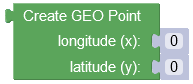where:

Argument                Description
`longitude (x)` Specifies the longitude coordinate of the geographic point. It represents the east-west position on the Earth's surface, measured in degrees.
`latitude (y)` Represents the latitude coordinate of the geographic point. It denotes the north-south position on the Earth's surface, measured in degrees.

Consider records in the following data table called `geolocation`: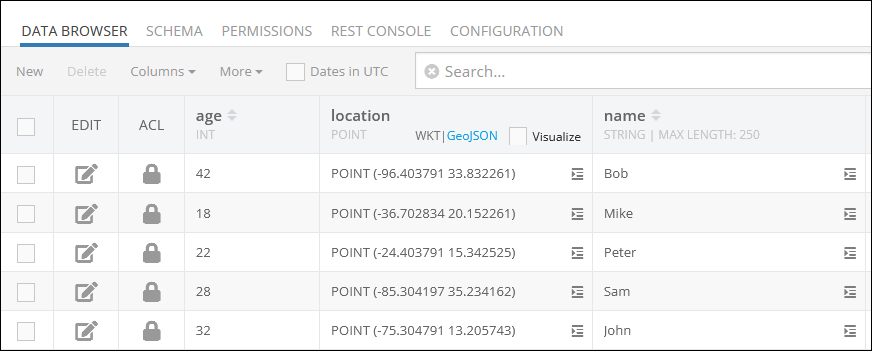Suppose you need to add a record to the data table presented above. The example below saves a new `GeoJSON` object(`POINT`) to the `geolocation` data table: As you can see, the Codeless block contains an object with the `location` property, that represents the column in the data table where `POINT` data objects are stored.where:

Argument                Description
`table name` Name of the data table where a new record must be saved.
`object` An object to save in the database. Object properties must match the names of the table columns. The object must not have the `objectId` property.
`return result` Optional parameter. When this box is checked, the operation returns the saved object with the `objectId` property.

After the Codeless logic runs, the operation adds a new object to the data table:In case you want to retrieve a specific object from the `geolocation` data table, refer to the Basic Object Retrieval topic to learn more about Codeless object retrieval operations.

## LINESTRING¶

The `LINESTRING` type is used to represent geometries composed of multiple `POINT` values with linear interpolation between each two consecutive points. The WKT representation of this type is:

``````LINESTRING (lon1 lat1, lon2 lat2, lon3 lat3, lon4 lat4)
``````

or

``````LINESTRING (x1 y1, x2 y2, x3 y3, x4 y4)
``````

for example, the `LINESTRING` below identifies the main stops of the historic Route 66:

``````LINESTRING (-87.52683788 41.85716752, -90.13875858 38.68967135, -95.93953983 36.2131248, -97.49959842 35.53656483, -101.8282117 35.26791494, -105.87118045 35.72083154, -106.61825076 35.14794417, -111.63900272 35.20182535, -118.24178592 34.07195769)
``````

The GeoJSON format for the `LINESTRING` values is:

``````{
"type": "LineString",
"coordinates": [
[
lon1 or x1,
lat1 or y1
],
[
lon2 or x2,
lat2 or x2
],
[
lon3 or x3,
lat3 or y3
],
[
lon4 or x4,
lat4 or y4
]
]
}
``````

Database values of this type are represented by the `BLLineString` class in the client application. The class provides access to the `Point` objects making up the linestring. The class has the constructors and methods as listed below. Notice that all `set` method return the current `LineString` object, which allows for convenient "chaining": `lineStringInstance.setPoints( value ).asWKT()`:

``````// creates a new instance of LineString
- (nonnull instancetype)initWithPoints:(NSArray<BLPoint *> * _Nonnull)points

// creates a LineString object from a WKT definition
+ (BLLineString * _Nullable)fromWkt:(NSString * _Nonnull)wkt;

// creates a LineString object from a GeoJSON definition
+ (BLLineString * _Nullable)fromGeoJson:(NSString * _Nonnull)geoJson;

// Objective-C approach is to use instance properties directly without get/set methods:

// returns a collection of Point objects making up this LineString
lineString.points;

// sets a collection of Point objects to define the LineString
lineString.points = ...;

// converts this LineString object into its WKT representation
- (NSString * _Nullable)asWkt

// converts this LineString object into its GeoJSON representation
- (NSDictionary<NSString *, id> * _Nullable)asGeoJson;
``````
``````// creates a new instance of LineString
public init(points: [BLPoint])
//e.g: let lineString = BLLineString(points: [BLPoint(x: 10, y: 10), BLPoint(x: 20, y: 20)])

// creates a LineString object from a WKT definition
public static func fromWkt(_ wkt: String) -> BLLineString?

// creates a LineString object from a GeoJSON definition
public static func fromGeoJson(_ geoJson: String) -> BLLineString?

// Swift approach is to use instance properties directly without get/set methods:

// returns a collection of Point objects making up this LineString
lineString.points

// sets a collection of Point objects to define the LineString
lineString.points = ...

// converts this LineString object into its WKT representation
public func asWkt() -> String?

// converts this LineString object into its GeoJSON representation
public func asGeoJson() -> [String : Any]?
``````

Consider the following example. The `Travel` table has an object identifying a travel route. The `route` column is of the `LINESTRING` type, its value is visualized in the map in the screenshot below: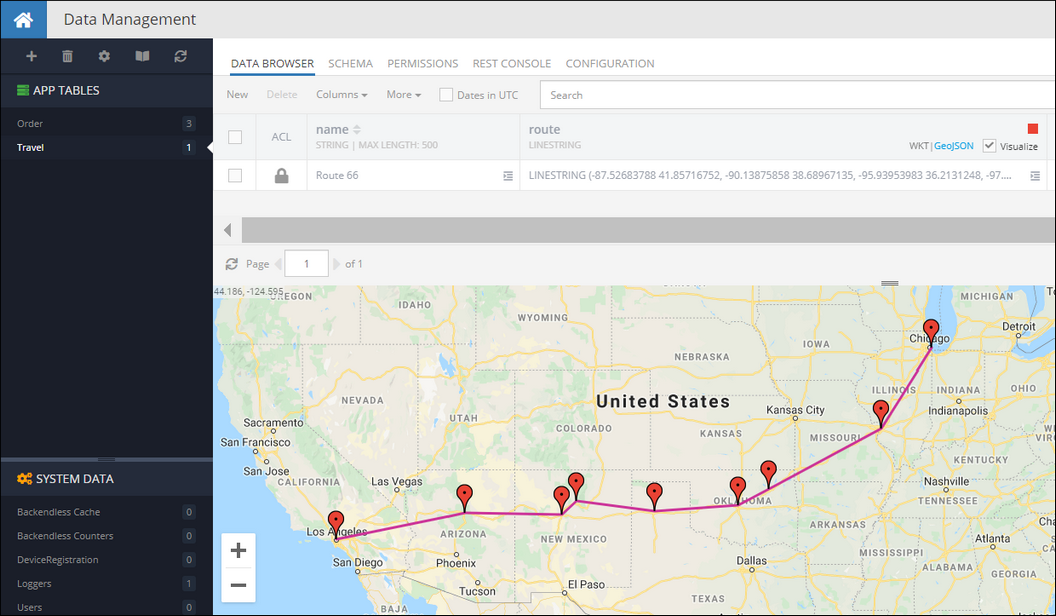The following code retrieves the `Travel` object from the table, gets its `route` property (which is a `LineString`) and accesses the points making up the linestring:

``````DataQueryBuilder *queryBuilder = [DataQueryBuilder new];
queryBuilder.whereClause = @"name = 'Route 66'";

[[Backendless.shared.data ofTable:@"Travel"] findWithQueryBuilder:queryBuilder responseHandler:^(NSArray *response) {
NSDictionary *travelRoute = response.firstObject;
NSString *routeName = travelRoute[@"name"];
BLLineString *routeDefinition = travelRoute[@"route"];
NSArray *points = routeDefinition.points;
} errorHandler:^(Fault *fault) {
NSLog(@"Error: %@", fault.message);
}];
``````
``````let queryBuilder = DataQueryBuilder()
queryBuilder.whereClause = "name = 'Route 66'"

Backendless.shared.data.ofTable("Travel").find(queryBuilder: queryBuilder, responseHandler: { response in
let travelRoute = response.first
let routeName = travelRoute?["name"] as? String
let routeDefinition = travelRoute?["route"] as? BLLineString
let points = routeDefinition?.points
}, errorHandler: { fault in
print("Error: \(fault.message ?? "")")
})
``````

Codeless Reference

The `LineString` data type is represented as a `GeoJSON` object in the Codeless.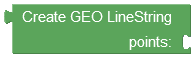where:

Argument                Description
`points` Represents a list containing Point objects. To populate the list, you must use the `"Create GEO Point"` Codeless block for each Point object you want to add.

Consider record in the following data table called `geolocation`:Suppose you need to add a new record to the data table presented above. In this context, the record to add represents a new destination between the `city1` and the `city2`, the destination itself is presented as the `LineString GeoJSON` object stored in the `destination` property.

In the provided example, a new LineString object is being saved to the `geolocation` data table. The `"Save Object"` Codeless block is utilized, containing an object with the `destination` property representing the column in the data table. Within this property, the `"Create GEO LineString"` Codeless block is used, which includes a list consisting of multiple instances of the `"Create GEO Point"` Codeless blocks.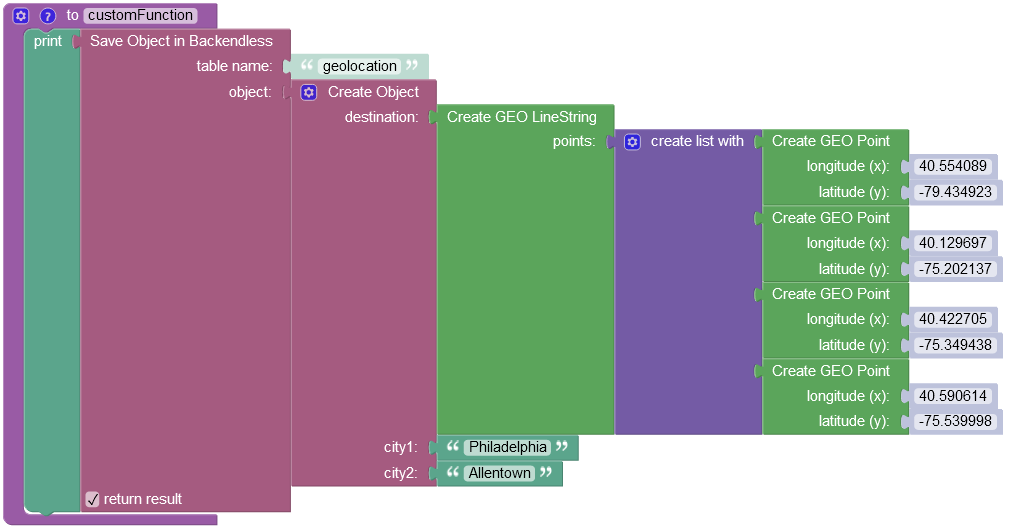where:

Argument                Description
`table name` Name of the data table where a new record must be saved.
`object` An object to save in the database. Object properties must match the names of the table columns. The object must not have the `objectId` property.
`return result` Optional parameter. When this box is checked, the operation returns the saved object with the `objectId` property.

After the Codeless logic runs, the operation adds a new object to the data table: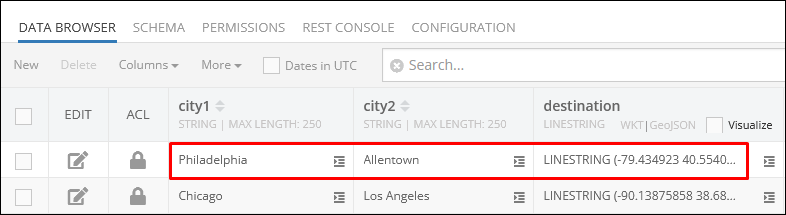In case you want to retrieve a specific object from the `geolocation` data table, refer to the Basic Object Retrieval topic to learn more about Codeless object retrieval operations.

## POLYGON¶

Value of the `POLYGON` type is a figure that is described by a number of `LINESTRING` values connected to form a single continuous exterior boundary. Additionally, a Polygon may  contain zero or more interior boundaries, where each interior boundary defines a hole in the Polygon. The WKT representation of this type is:

``````POLYGON ((lon1 lat1, lon2 lat2, lon3 lat3),
(hole-lon1 hole-lat1, hole-lon2 hole-lat2, hole-lon3 hole-lat3),
(...),(...))
``````

or

``````POLYGON ((x1 y1, x2 y2, x3 y3),
(hole-x1 hole-y1, hole-x2 hole-y2, hole-x3 hole-y3),
(...),(...))
``````

where the first group of coordinates defines the exterior boundary and all subsequent groups defines the holes. The first group is mandatory.

for example, the `POLYGON` below identifies the outline of The Pentagon - the US Department of Defense headquarters. It also includes a hole - which is the inner plaza.

``````POLYGON ((-77.05781934 38.87248788,
-77.05474017 38.87287211,
-77.0533025 38.8706001,
-77.05556629 38.86883758,
-77.05848453 38.87002374,
-77.05781934 38.87248788),
(-77.05669282 38.87156906,
-77.05551265 38.87170271,
-77.05494402 38.8708507,
-77.05577014 38.87030775,
-77.05688594 38.87074211,
-77.05669282 38.87156906))
``````

The GeoJSON format for the `POLYGON` values is:

``````{
"type": "Polygon",
"coordinates": [
[
[
lon1,
lat1
],
[
lon2,
lat2
],
[
lon3,
lat3
]
],
[
[
hole-lon1,
hole-lat1
],
[
hole-lon2,
hole-lat2
],
[
hole-lon3,
hole-lat3
]
]
]
}
``````

Database values of this type are represented by the `BLPolygon` class in the client application. The class provides access to the `LineString` objects making up the polygon. The class has the constructors and methods as listed below. Notice that all `set` method return the current Polygon object, which allows for convenient "chaining" for property assignments: `polygonInstance.setBoundary( value ).asWKT()`:

``````// creates a new instance of Polygon (holes are optional)
- (nonnull instancetype)initWithBoundary:(BLLineString * _Nonnull)boundary holes:(BLLineString * _Nullable)holes;

// creates a Polygon object from a WKT definition
+ (BLPolygon * _Nullable)fromWkt:(NSString * _Nonnull)wkt;

// creates a Polygon object from a GeoJSON definition
+ (BLPolygon * _Nullable)fromGeoJson:(NSString * _Nonnull)geoJson;

// returns a LineString which defines the external boundary of the polygon
polygon.boundary;

// returns a collection of LineString objects each identifying a hole
polygon.holes;

// converts this Polygon object into its WKT representation
- (NSString * _Nullable)asWkt;

// converts this Polygon object into its GeoJSON representation
- (NSDictionary<NSString *, id> * _Nullable)asGeoJson;
``````
``````// creates a new instance of Polygon (holes are optional)
public init(boundary: BLLineString, holes: BLLineString?)
// e.g.: let polygon = BLPolygon(boundary: BLLineString(points: // array of BLPoints), holes: nil)

// creates a Polygon object from a WKT definition
public static func fromWkt(_ wkt: String) -> BLLineString?

// creates a Polygon object from a GeoJSON definition
public static func fromGeoJson(_ geoJson: String) -> BLLineString?

// returns a LineString which defines the external boundary of the polygon
polygon.boundary

// returns a collection of LineString objects each identifying a hole
polygon.holes

// converts this Polygon object into its WKT representation
public func asWkt() -> String?

// converts this Polygon object into its GeoJSON representation
public func asGeoJson() -> [String : Any]?
``````

Consider the following example. The `Building` table has an object identifying a shape of a building. The `shape` column is of the `POLYGON` type, its value is visualized in the map in the screenshot below: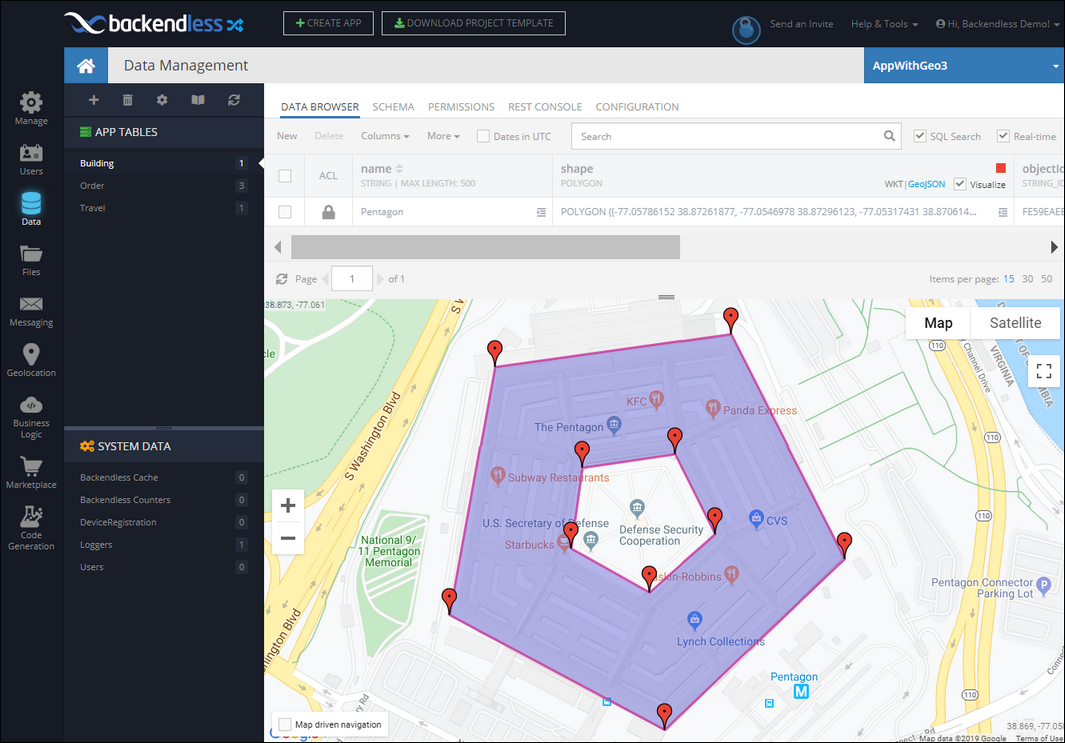The following code retrieves the `Building` object from the table, gets its `shape` property (which is a `Polygon`) and accesses its external boundary and the hole.

``````DataQueryBuilder *queryBuilder = [DataQueryBuilder new];
queryBuilder.whereClause = @"name = 'Pentagon'";

[[Backendless.shared.data ofTable:@"Building"] findWithQueryBuilder:queryBuilder responseHandler:^(NSArray *response) {
NSDictionary *building = response.firstObject;
NSString *buildingName = building[@"name"];
BLPolygon *buildingShape = building[@"shape"];
BLLineString *externalBoundary = buildingShape.boundary;
BLLineString *holes = buildingShape.holes;
} errorHandler:^(Fault *fault) {
NSLog(@"Error: %@", fault.message);
}];
``````
``````let queryBuilder = DataQueryBuilder()
queryBuilder.whereClause = "name = 'Pentagon'"

Backendless.shared.data.ofTable("Building").find(queryBuilder: queryBuilder, responseHandler: { response in
let building = response.first
let buildingName = building?["name"] as? String
let buildingShape = building?["shape"] as? BLPolygon
let externalBoundary = buildingShape?.boundary
let holes = buildingShape?.holes
}, errorHandler: { fault in
print("Error: \(fault.message ?? "")")
})
``````

Codeless Reference

The `Polygon` data type is represented as a `GeoJSON` object in the Codeless. This object consists of two properties: `boundary` and `holes`: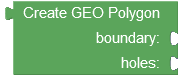where:

Argument                Description
`boundary` Represents the outer boundary of the polygon. It defines the primary shape or contour that encloses the entire area of the polygon. This boundary forms the outermost shape that encompasses the entire polygon area.

Must be a list containing Point objects. To populate the list, you must use the `"Create GEO Point"` Codeless block for each Point object you want to add.
`holes` Represents the inner boundaries of the polygon. It specifies additional shapes or contours that are located within the outer boundary of the polygon. These holes create empty regions or cutouts within the main polygon area. Each hole defines a distinct interior area that is excluded from the polygon's total coverage.

Must be a list containing the LineString objects populated using the `"Create GEO LineString"` Codeless block. Each LineString object must also contain a list of Point objects created using the `"Create GEO Point"` Codeless block.

Consider the structure of the following data table called `geolocation`: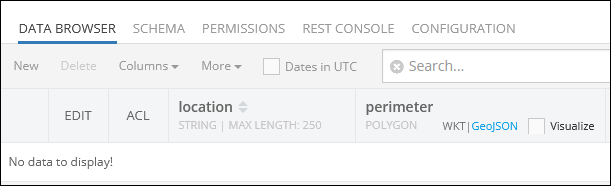Suppose you need to add a simple polygon(without interior boundaries) to the data table.

Important

The Codeless logic below represents a quadrilateral polygon, which is a geometrical figure with four sides. To create a polygon, the list containing Point objects must have the same longitude and latitude values in both the first and the last objects. The last object is needed to enclose the polygon, completing its shape.

The example below saves a new `GeoJSON` object(`Polygon`) to the `geolocation` data table: As you can see, the Codeless block contains an object with the `perimeter` property, that represents the column in the data table where the `Polygon` data objects are stored. The saved object represents the perimeter of the `Chicago` city.where:

Argument                Description
`table name` Name of the data table where a new record must be saved.
`object` An object to save in the database. Object properties must match the names of the table columns. The object must not have the `objectId` property.
`return result` Optional parameter. When this box is checked, the operation returns the saved object with the `objectId` property.

After the Codeless logic runs, the operation adds a new object to the data table: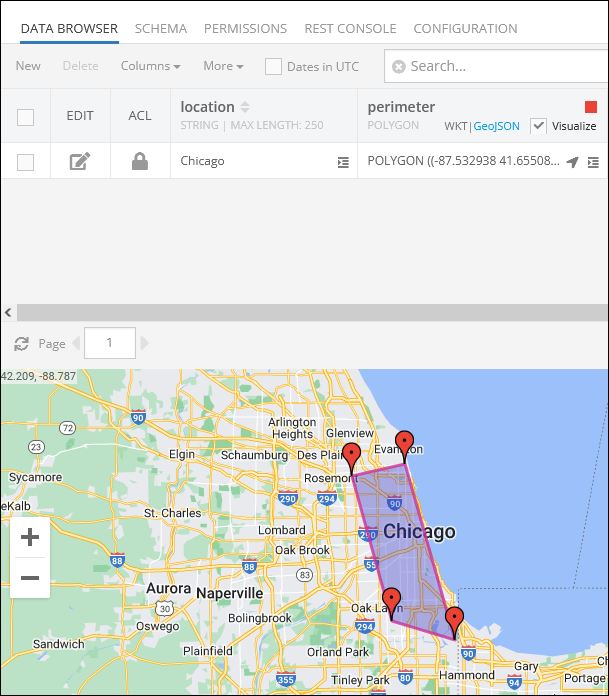In case you need to add a polygon that contains the interior boundaries, you have to utilize the `holes` parameter, adding the list containing the LineString objects where each LineString object must also contain a list of Point objects created using the `"Create GEO Point"` Codeless block.

The coordinates of the interior boundary must be reversed against the exterior boundary. So the first `Point` of the interior boundary must start closer to the last `Point` of the exterior boundary: Consider the following screenshot for better explanation:To draw the geometric figure highlighted above, the following Codeless logic is used: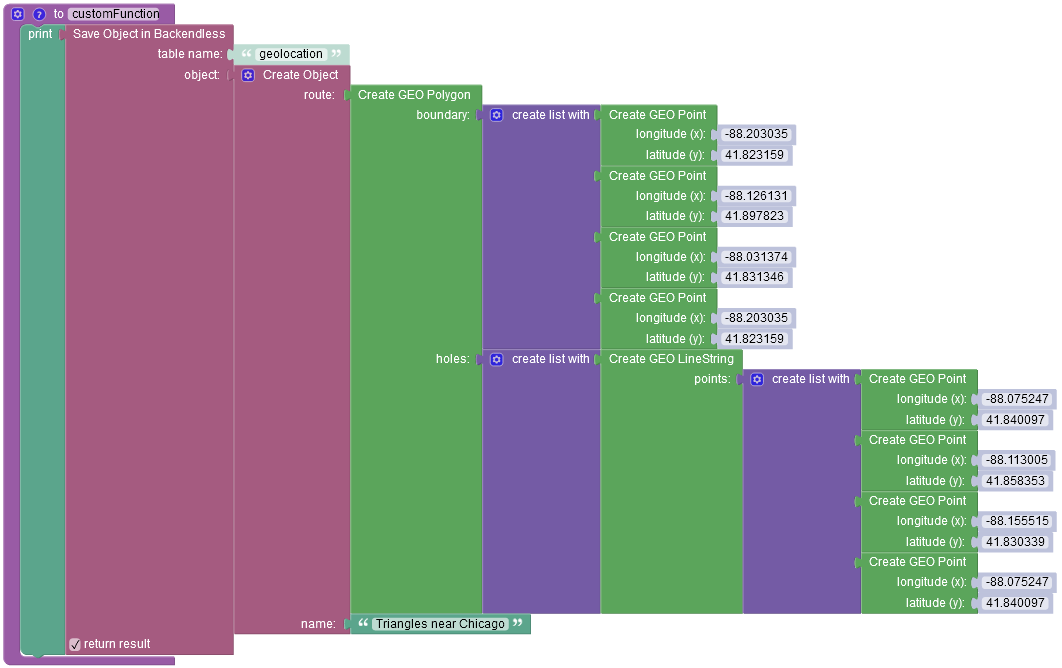After the Codeless logic runs, the operation adds a new object to the data table: